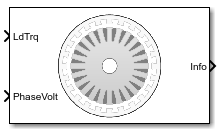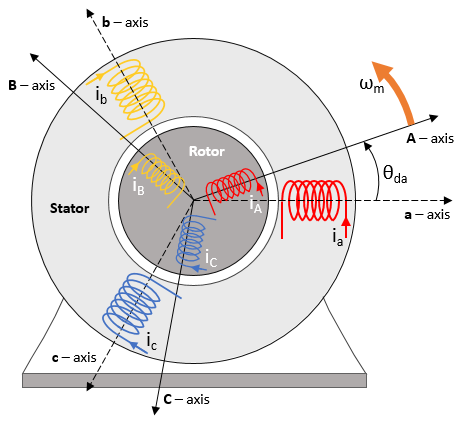Induction Motor

Three-phase induction motor

• Library:
• Powertrain Blockset / Propulsion / Electric Motors and Inverters

Motor Control Blockset / Electrical Systems / Motors

•Description

The Induction Motor block implements a three-phase induction motor. The block uses the three-phase input voltages to regulate the individual phase currents, allowing control of the motor torque or speed.

By default, the block sets the Simulation Type parameter to Continuous to use a continuous sample time during simulation. If you want to generate code for fixed-step double- and single-precision targets, considering setting the parameter to Discrete. Then specify a Sample Time, Ts parameter.

Three-Phase Sinusoidal Model Electrical System

The block implements equations that are expressed in a stationary rotor reference (qd) frame. The d-axis aligns with the a-axis. All quantities in the rotor reference frame are referred to the stator.The block uses these equations to calculate the electrical speed (ωem) and slip speed (ωslip).

To calculate the dq rotor electrical speed with respect to the rotor A-axis (dA), the block uses the difference between the stator a-axis (da) speed and slip speed:

To simplify the equations for the flux, voltage, and current transformations, the block uses a stationary reference frame:

$\begin{array}{l}{\omega }_{da}=0\\ {\omega }_{dA}=-{\omega }_{em}\end{array}$

CalculationEquation
Flux

Current

Inductance

Electromagnetic torque

Power invariant dq transformation to ensure that the dq and three phase powers are equal

The equations use these variables.

 ωm Angular velocity of the rotor (rad/s) ωem Electrical rotor speed (rad/s) ωslip Electrical rotor slip speed (rad/s) ωsyn Synchronous rotor speed (rad/s) ωda dq stator electrical speed with respect to the rotor a-axis (rad/s) ωdA dq stator electrical speed with respect to the rotor A-axis (rad/s) Θda dq stator electrical angle with respect to the rotor a-axis (rad) ΘdA dq stator electrical angle with respect to the rotor A-axis (rad) Lq, Ld q- and d-axis inductances (H) Ls Stator inductance (H) Lr Rotor inductance (H) Lm Magnetizing inductance (H) Lls Stator leakage inductance (H) Llr Rotor leakage inductance (H) vsq, vsd Stator q- and d-axis voltages (V) isq, isd Stator q- and d-axis currents (A) λsq, λsd Stator q- and d-axis flux (Wb) irq, ird Rotor q- and d-axis currents (A) λrq, λrd Rotor q- and d-axis flux (Wb) va, vb, vc Stator voltage phases a, b, c (V) ia, ib, ic Stator currents phases a, b, c (A) Rs Resistance of the stator windings (Ohm) Rr Resistance of the rotor windings (Ohm) P Number of pole pairs Te Electromagnetic torque (Nm)

Mechanical System

The motor angular velocity is given by:

$\begin{array}{c}\frac{d}{dt}{\omega }_{m}=\frac{1}{J}\left({T}_{e}-{T}_{f}-F{\omega }_{m}-{T}_{m}\right)\\ \frac{d{\theta }_{m}}{dt}={\omega }_{m}\end{array}$

The equations use these variables.

 J Combined inertia of motor and load (kgm^2) F Combined viscous friction of motor and load (N·m/(rad/s)) θm Motor mechanical angular position (rad) Tm Motor shaft torque (Nm) Te Electromagnetic torque (Nm) Tf Motor shaft static friction torque (Nm) ωm Angular mechanical velocity of the motor (rad/s)

Power Accounting

For the power accounting, the block implements these equations.

Bus Signal DescriptionVariableEquations

PwrInfo

PwrTrnsfrd — Power transferred between blocks

• Positive signals indicate flow into block

• Negative signals indicate flow out of block

PwrMtr

Mechanical power

Pmot

PwrBus

Electrical power

Pbus

PwrNotTrnsfrd — Power crossing the block boundary, but not transferred

• Positive signals indicate an input

• Negative signals indicate a loss

PwrElecLoss

Resistive power loss

Pelec

PwrMechLoss

Mechanical power loss

Pmech

When Port Configuration is set to Torque:

When Port Configuration is set to Speed:

PwrStored — Stored energy rate of change

• Positive signals indicate an increase

• Negative signals indicate a decrease

PwrMtrStored

Stored motor power

Pstr

The equations use these variables.

 Rs Stator resistance (Ohm) Rr Motor resistance (Ohm) ia, ib, ic Stator phase a, b, and c current (A) isq, isd Stator q- and d-axis currents (A) van, vbn, vcn Stator phase a, b, and c voltage (V) ωm Angular mechanical velocity of the rotor (rad/s) F Combined motor and load viscous damping (N·m/(rad/s)) Te Electromagnetic torque (Nm) Tf Combined motor and load friction torque (Nm)

Ports

Input

expand all

Load torque on the motor shaft, Tm, in N·m.

Dependencies

To create this port, select Torque for the Port configuration parameter.

Angular velocity of the rotor, ωm, in rad/s.

Dependencies

To create this port, select Speed for the Port configuration parameter.

Stator terminal voltages, Va, Vb, and Vc, in V.

Output

expand all

The bus signal contains these block calculations.

Signal DescriptionVariableUnits

IaStator

Stator phase current A

ia

A

IbStator

Stator phase current B

ib

A

IcStator

Stator phase current C

ic

A

IdSync

Direct axis current

id

A

IqSync

iq

A

VdSync

Direct axis voltage

vd

V

VqSync

vq

V

MtrSpd

Angular mechanical velocity of the rotor

ωm

MtrMechPos

Rotor mechanical angular position

θm

MtrPos

Rotor electrical angular position

θe

MtrTrq

Electromagnetic torque

Te

N·m

PwrInfo

PwrTrnsfrd

PwrMtr

Mechanical power

Pmot

W
PwrBus

Electrical power

Pbus

W

PwrNotTrnsfrd

PwrElecLoss

Resistive power loss

Pelec

W
PwrMechLoss

Mechanical power loss

Pmech

W

PwrStored

PwrMtrStored

Stored motor power

Pstr

W

Phase a, b, c current, ia, ib, and ic, in A.

Motor torque, Tmtr, in N·m.

Dependencies

To create this port, select Speed for the Port configuration parameter.

Angular speed of the motor, ωmtr, in rad/s.

Dependencies

To create this port, select Torque for the Port configuration parameter.

Parameters

expand all

Block Options

By default, the block uses a continuous sample time during simulation. If you want to generate code for single-precision targets, considering setting the parameter to Discrete.

Dependencies

Setting Simulation Type to Discrete creates the Sample Time, Ts parameter.

Integration sample time for discrete simulation, in s.

Dependencies

Setting Simulation Type to Discrete creates the Sample Time, Ts parameter.

This table summarizes the port configurations.

Port ConfigurationCreates Input PortCreates Output Port

Torque

LdTrq

MtrSpd

Speed

Spd

MtrTrq

Parameters

Motor pole pairs, P.

Stator resistance, RS, in ohms and leakage inductance, Lls, in H.

Rotor resistance, Rr, in ohms and leakage inductance, Llr, in H.

Magnetizing inductance, Lm, in H.

Mechanical properties of the rotor:

• Inertia, J, in kg·m^2

• Viscous damping, F, in N·m/(rad/s)

• Static friction, Tf, in N·m

Dependencies

To enable this parameter, select Torque for the Port configuration.

Initial Values

Initial rotor angular position, θm0, in rad.

Initial angular velocity of the rotor, ωm0, in rad/s.

Dependencies

To enable this parameter, select Torque for the Port configuration.

 Mohan, Ned. Advanced Electric Drives: Analysis, Control and Modeling Using Simulink. Minneapolis, MN: MNPERE, 2001.

Powertrain Blockset DocumentationGet trial now